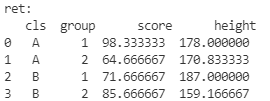﻿ Pandas分组聚合之groupby()、agg()方法的使用教程_python_脚本之家
python# Pandas分组聚合之groupby()、agg()方法的使用教程

## 创建一个dataframe结构

```import pandas as pd

df = pd.DataFrame(
data={
'name': ['z_s', 'l_s', 'w_w', 'z_l', 'y_s', 'j_j', 'l_b', 'z_f', 'hs_q', 'lbl_k', 'qy_n', 'mg_n'],
'score': [100, 97, 98, 89, 67, 59, 29, 87, 78, 89, 88, 80],
'group': [1, 1, 1, 1, 1, 1, 2, 2, 2, 2, 2, 2],
'cls': ['A', 'A', 'A', 'B', 'B', 'B', 'A', 'A', 'A', 'B', 'B', 'B'],
'height': [178.0, 180.0, 176.0, 182.0, 189.0, 190.0, 172.5, 175.0, 165.0, 160.0, 158.5, 159.0]
},
index=['stu_' + str(i) for i in np.arange(1, 13, 1)]
)
print('df:\n', df)
```## 分组函数 groupby()

### 初识分组聚合

```# 先按照班级进行分组，再统计各个组里面的成绩的最大值！
ret = df.groupby(by=['cls'])['score'].max().reset_index()
print('ret:\n', ret)
``````ret = df.groupby(by=['cls'])	# 将数据以 cls 进行分组，返回 DataFrameGroupBy 对象
print(ret)		# <pandas.core.groupby.generic.DataFrameGroupBy object at 0x000002B6B2003A60>
ret = ret['score']				# 取出 score 列，返回 SeriesGroupBy 对象
print(ret)		# <pandas.core.groupby.generic.SeriesGroupBy object at 0x000002B6B59EAFD0>
ret = ret.max()					# 取出 score 中的最大值，返回 Series 对象
print(ret)
"""
cls
A    100
B     89
Name: score, dtype: int64
"""
ret = ret.reset_index()			# 重设索引，返回 DataFrame 对象
print(ret)
"""
cls  score
0   A    100
1   B     89
"""
```

### 多重行索引分组聚合

```# 先按照班级分组，再按照小组分组，最后统计各个小组内成绩的最大值
ret = df.groupby(by=['cls', 'group'])['score'].max()
print('ret:\n', ret)
print('index:\n', ret.index)	# MultiIndex ---多重行索引
ret = ret.reset_index()			# 重设索引
print(ret)
```### 对多列数据进行分组聚合

```# 按照班级分组，统计各个组内 成绩、身高的平均值
ret = df.groupby(by=['cls'])[['score', 'height']].mean().reset_index()
print('ret:\n', ret)
```### 综合应用

```# 先按照班级分组、再按照小组分组---统计各个小组内的成绩的平均值、身高的平均值
ret = df.groupby(by=['cls', 'group'])[['score', 'height']].mean().reset_index()
print('ret:\n', ret)
```## 聚合函数 agg（aggregate）

### 求 多列数据 的 多个指标

```# 使用agg 方法 可以对多列数据一次性求出多个指标
ret = df.loc[:, ['score', 'height']].agg([np.max, np.mean])
print('ret:\n', ret)
```### 对多列数据统计不同的指标

```ret = df.agg({'score': [np.mean], 'height': [np.max]})
print('ret:\n',ret)
```### 对多列数据统计不同个数的指标

```ret = df.agg({'score': [np.mean, np.max, np.median], 'height': [np.mean]})
print('ret:\n', ret)
``````ret = df.groupby(by=['cls']).agg({'height': [np.max,np.mean], 'score': [np.min]})
print('ret:\n', ret)
```### agg调用 自定义函数

```ret = df.loc[:, 'score'].agg(lambda x: x + 1)
print('ret1:\n', ret)

return x + 1

print('ret2:\n', ret)

# 对多列 使用自定义函数
print('ret3:\n', ret)
```### 使用agg 调用numpy的统计指标

```# 统计所有同学成绩的和
ret = df.loc[:, 'score'].agg(np.sum)
print('ret:\n',ret)
print('type:\n',type(ret))

# # 统计所有同学 成绩以及身高 的和
ret = df.loc[:, ['score', 'height']].agg(np.sum)
print('ret:\n', ret)
print('type:\n',type(ret))

# 统计身高 + 成绩（无意义的，只是为了演示能够 同一行相加）
ret = df.loc[:, ['score', 'height']].agg(np.sum, axis=1)	# 使用axis指定相加的方向
print('ret:\n', ret)
print('type:\n',type(ret))
```